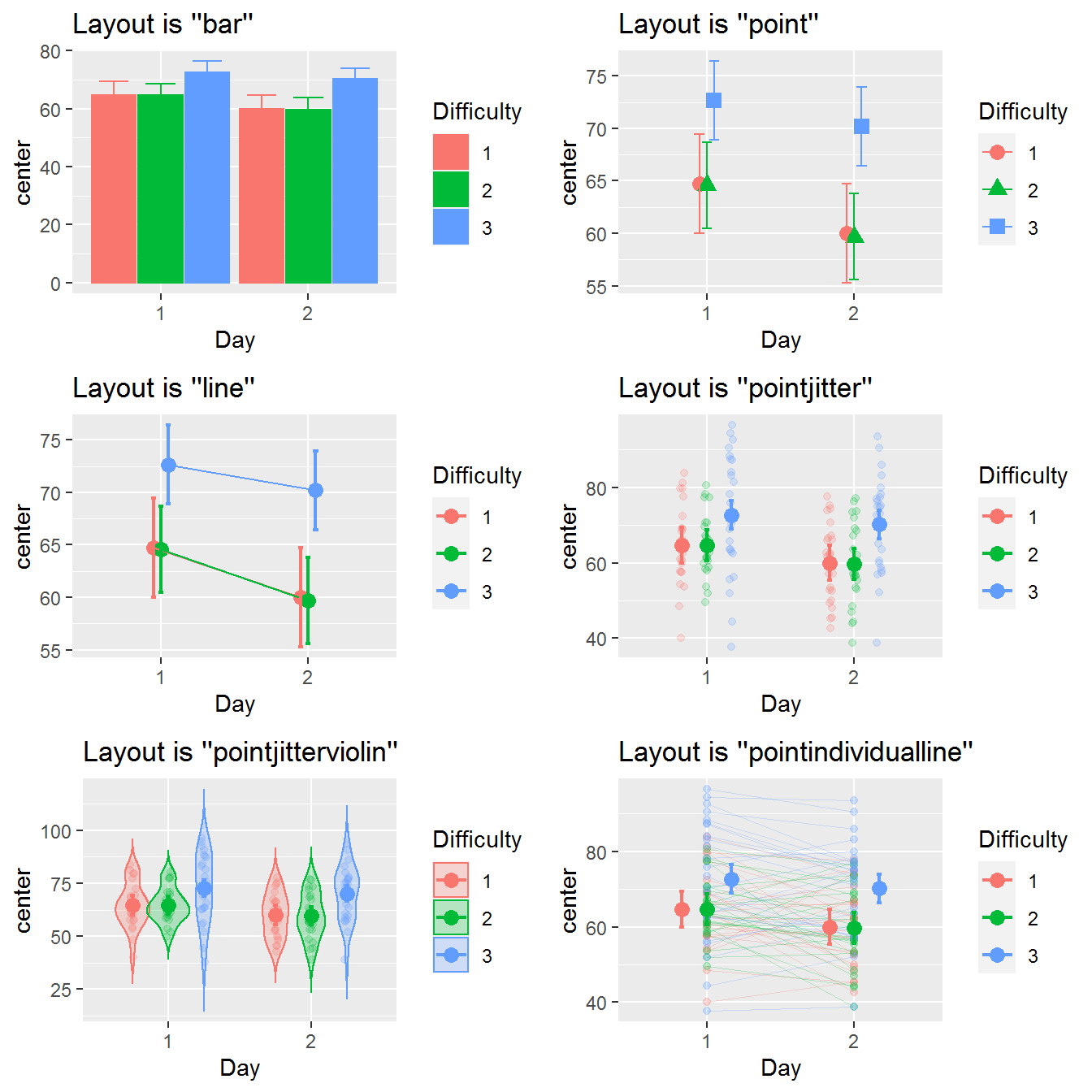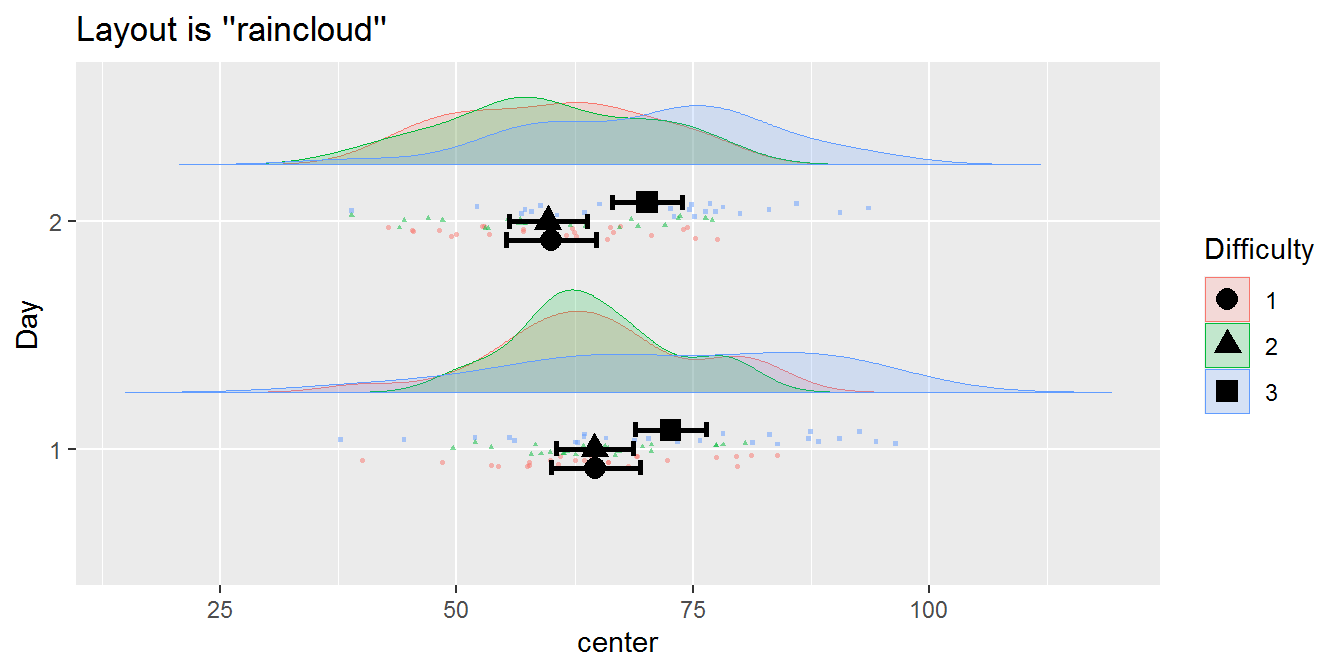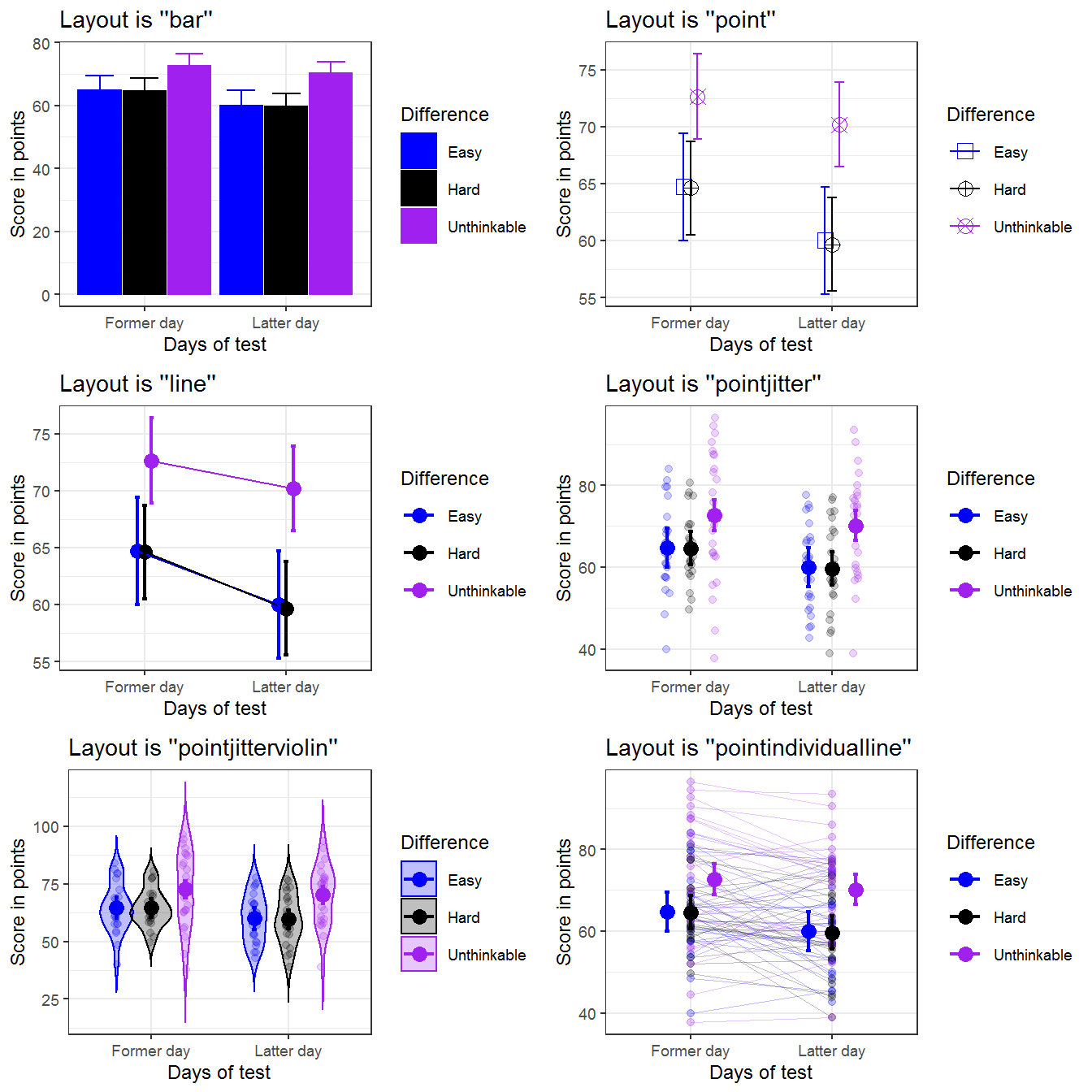superbPlot() comes with seven built-in layouts for plotting your data. However, it is possible to add additional, custom-made layouts. In this vignette, we present rapidly the existing layouts, then show how to supplement superb with your own layouts.

## The built-in plot layouts

When calling superbPlot(), you use the plotStyle = "layout" option to indicate which layout you wish to use. Internally, superbPlot() is calling a function whose name is superbPlot."layout"(). For example, with plotStyle = "line", the plot is actually performed by the function superbPlot.line().

The seven layout available in superbPlot package are :

• superbPlot.line() : shows the results as points and lines,

• superbPlot.point(): shows the results as points only,

• superbPlot.bar() : shows the results using bars,

• superbPlot.pointjitter(): shows the results with points, and the raw data with jittered points,

• superbPlot.pointjitterviolin(): also shows violin plot behind the jitter points, and

• superbPlot.pointindividualline(): show the results with fat points, and individual results with thin lines,

• superbPlot.raincloud(): Shows the results with distribution and jitter.

To determine if a certain function is superbPlot-compatible, use the following function:

superb:::is.superbPlot.function("superbPlot.line")
##  TRUE

where you put between quote the name of a function. When devising your own, custom-made function, it is a good thing to check that it is superbPlot-compatible.

## Illustrating the built-in layouts

To get a sense of the currently available layouts, we first generate a dataset composed of randomly generated scores mimicking a 3 $$\times$$ 2 design with three degrees of Difficulties (as a between-group factor) and two days of testing (as a within-subject factor). It is believed (and simulated) that all two factors have main effets on the scores.

testdata <- GRD(
RenameDV   = "score",
SubjectsPerGroup = 25,
BSFactors  = "Difficulty(3)",
WSFactors  = "Day(day1, day2)",
Population = list(mean = 65,stddev = 12,rho = 0.5),
Effects    = list("Day" = slope(-5), "Difficulty" = slope(3) )
)
head(testdata)
##   id Difficulty score.day1 score.day2
## 1  1          1   60.78673   57.04693
## 2  2          1   66.00436   65.90878
## 3  3          1   77.47237   66.24295
## 4  4          1   60.98000   52.86207
## 5  5          1   64.23464   42.72996
## 6  6          1   72.32137   62.69619

For simplicity, we define a function whose arguments are the dataset and the layout:

mp <- function(data, style, ...) {
superbPlot(data,
WSFactors = "Day(2)",
BSFactors = "Difficulty",
variables = c("score.day1", "score.day2"),
plotStyle = style,
...
)+labs(title = paste("Layout is ''",style,"''",sep=""))
}

Lets compute the plots will the first six built-in layouts and show them

p1 <- mp(testdata, "bar")
p2 <- mp(testdata, "point")
p3 <- mp(testdata, "line")
p4 <- mp(testdata, "pointjitter" )
p5 <- mp(testdata, "pointjitterviolin")
p6 <- mp(testdata, "pointindividualline")

library(gridExtra)
grid.arrange(p1,p2,p3,p4,p5,p6,ncol=2)Figure 1a. Look of the six built-in layouts on the same random dataset

The last format, a raincloud plot (Allen et al., 2021), is better seen with coordinates flipped over:

mp(testdata, "raincloud") + coord_flip()Figure 1b. The seventh layout, the raincloud

For more controls, you can manually set the colors, the fills and/or the shapes, as done here in a list:

ornate = list(
scale_colour_manual( name = "Difference",
labels = c("Easy", "Hard", "Unthinkable"),
values = c("blue", "black", "purple")) ,
scale_fill_manual( name = "Difference",
labels = c("Easy", "Hard", "Unthinkable"),
values = c("blue", "black", "purple")) ,
scale_shape_manual( name = "Difference",
labels = c("Easy", "Hard", "Unthinkable") ,
values = c(0, 10, 13)) ,
theme_bw(base_size = 9) ,
labs(x = "Days of test", y = "Score in points" ),
scale_x_discrete(labels=c("1" = "Former day", "2" = "Latter day"))
)
library(gridExtra)
grid.arrange(
p1+ornate, p2+ornate, p3+ornate,
p4+ornate, p5+ornate, p6+ornate,
ncol=2)Figure 2a. The six built-in template with ornamental styling added.

These are just a few examples. However, if these layouts do not fit yours needs, it is possible to devise a custom-made layout and inform superbPlot to use it. To that end, see the instructions below.

## Devising a custom-made plot layout

In a nutshell, the purpose of superbPlot() is to

• compile the summary information (location of the summary statistic, upper width and lower width of the interval) and that, for each level of the factors;

• applies all the adjustments needed in producing the summary;

• and finally, calls a plot function accepting pre-defined arguments

In devising your own plot function, it is important that (i) the function name begins with superbPlot.; (ii) the function accept very specific arguments with very precise names.

Here is the header for a function corresponding to a plot style called, say, foo (plotStyle = "foo"):

superbPlot.foo <- function(
summarydata,
xfactor,
groupingfactor,
rawdata
# any optional argument you wish
) {
plot <- ggplot() ## ggplot instructions...
return(plot)
}

In what follow, it is assumed that one factor is placed on the horizontal axis (xfactor), another one is used to group the point (groupingfactor), and up to two additional factors will results in columns and rows of panels (addfactors; of course, in devising your own template, you may use different placement). superbPlot() is restricted to a maximum of four factors.

The arguments are:

• summarydata: this data frame will contain the column center indicating the statistic’s value, lowerwidth and upperwidth indicating how many units below and above center the error bar extends. The data frame will also have columns for all the factors, and there will be as many lines as there are combinations of factors.

• xfactor is the factor to put on the horizontal axis;

• groupingfactor is the factor used to create groups of points;

• addfactors are up to two additional factors to create the rows and columns of panels. addfactors is formatted for facetting (e.g., for factors “A” and “B,” addfactors would be “A~B”);

• rawdata : this data.frame contains the raw data with factors being transformed as.factor and the dependent column being renamed DV. When the data are in wide format, rawdata is reshaped to long format.

• {optional arguments} can be used. They must be named here; when calling superbPlot(), any argument whose name match your optional argument will be transmitted to your custom-made function.

## The simplest example

What follow is a simple example that will design a template that we will call simple. This layout will display the descriptive statistics and error bars. Everything will be black and white (no color instruction) and superimposed (no grouping instruction).

The result will be: